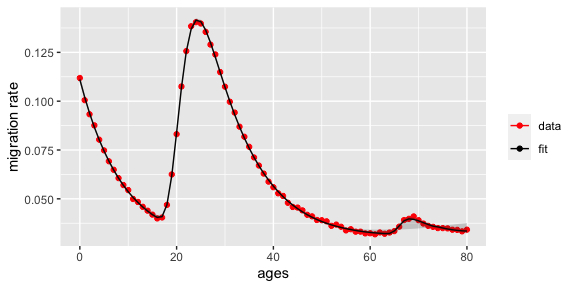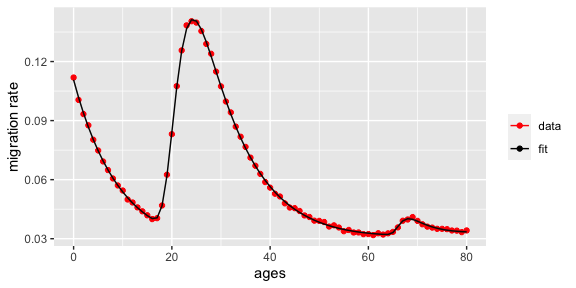# Model Convergence With mig_estimate_rc

## Model Diagnostics in Bayesian Models

### Overview

The mig_estimate_rc function estimates Rogers-Castro parameters in a Bayesian framework using a Markov Chain Monte Carlo (MCMC) algorithm, via the Stan programming language (Carpenter et al. 2017). This method of estimation is advantageous for models that are difficult to estimate and highly non-linear (such as the Rogers-Castro model). However for models estimated using MCMC algorithms, it is necessary to check the model diagnostics before interpreting model results.

The output of mig_estimate_rc allows you to easily check two diagnostic values in the check_converge object of the output:

• The potential scale reduction statistic, commonly referred to as the R-hat statistic, provides insight into whether the model has converged (Gelman, Rubin, and others 1992). You want the R-hat values to be close to 1, and R-hat values far greater than 1 indicate that convergence has not been achieved. Generally, Gelman et al. recommend ensuring that R-hat values are below 1.1, although there is no universally agreed upon threshold (Gelman et al. 2013).

• The Effective sample size provides insight into the autocorrelation among samples in the same chain. The larger the effective sample size the better. If your effective sample size is small, you should consider increasing the number of MCMC samples.

The rest of this document focuses on dealing with convergence issues, which will likely be reflected as large R-hat values. It is not uncommon to find that your model has not converged. This is particularly true if you are fitting a the Poisson model (i.e., providing count data) with a large total population size and/or you are fitting either the 11 or 13 parameter models. Resolving a non-convergent model is not always straight-forward, but we will explain some strategies that can be used with mig_estimate_rc.

## Example of a Non-Convergent Model

Here is an example of a non-convergent model. We will be fitting the full 13-parameter model. In this example, we provide count data meaning that we are fitting the Poisson model.

library(rcbayes)
library(tibble)
library(ggplot2)

ages <- 0:80
migrants <- c(11804, 10606, 9845, 9244, 8471, 7940, 7348, 6885, 6431,
6055, 5454, 4997, 4845, 4596, 4397, 4814, 4592, 4646, 5386,
7180, 11374, 14713, 17195, 18937, 19223, 19091, 18507,
17615, 16929, 15693, 15246, 14152, 13365, 12340, 11609,
10278, 9547, 8992, 8438, 7883, 7315, 6909, 6730, 6272,
5994, 6087, 5896, 5592, 5487, 5237, 6021, 5933, 5577,
5674, 5503, 4916, 5008, 4822, 4824, 4696, 4086, 4019,
4139, 4054, 4134, 3625, 3871, 4238, 4306, 4440, 3118,
2980, 2885, 2845, 2795, 2085, 2076, 2035, 2030, 1986, 2037)
pop <- c(105505, 105505, 105505, 105505, 105505, 106126, 106126, 106126,
106126, 106126, 100104, 100104, 100104, 100104, 100104, 114880,
114880, 114880, 114880, 114880, 136845, 136845, 136845, 136845,
136845, 136582, 136582, 136582, 136582, 136582, 141935, 141935,
141935, 141935, 141935, 134097, 134097, 134097, 134097, 134097,
130769, 130769, 130769, 130769, 130769, 133718, 133718, 133718,
133718, 133718, 154178, 154178, 154178, 154178, 154178, 145386,
145386, 145386, 145386, 145386, 126270, 126270, 126270, 126270,
126270, 108314, 108314, 108314, 108314, 108314, 79827, 79827,
79827, 79827, 79827, 59556, 59556, 59556, 59556, 59556, 59556)
rc_res <- mig_estimate_rc(
ages, migrants, pop,
pre_working_age = TRUE,
working_age = TRUE,
retirement = TRUE,
post_retirement = TRUE
)

The check_converge object in the function output will provide model diagnostics.

rc_res[['check_converge']]
##                    mean     se_mean    n_eff     Rhat
## alpha1   0.113605451 0.007795931 2.157746 3.707907
## alpha2   0.111764533 0.007978197 2.182820 3.439780
## alpha3   0.200212843 0.047664167 2.427855 2.333646
## a1       0.086013430 0.002929845 2.444840 2.366614
## a2       0.206149089 0.004343064 2.627664 2.083178
## a3       0.011611407 0.004653543 2.090140 4.707573
## a4       0.011160726 0.002049349 7.114026 1.218068
## mu2     21.190347982 0.110825508 2.426008 2.405560
## mu3     65.882454118 0.527963547 2.994252 1.736682
## lambda2  0.391006779 0.007558619 2.985018 1.751782
## lambda3  0.564868093 0.211502782 2.350907 2.662197
## lambda4  0.002918706 0.007446659 2.968181 1.909460
## c           0.014062420 0.005355023 2.761192 1.922253

We see that there are many R-hat values that are very large and thus the model has not converged properly. Even if we were to continue to plot the results, we can see that bounds don’t look quite right.

rc_res[["fit_df"]] %>%
ggplot(aes(ages, data)) +
geom_point(aes(color = "data")) +
geom_line(aes(x = ages, y = median, color = "fit")) +
geom_ribbon(aes(ymin = lower, ymax = upper), alpha = 0.2) +
scale_color_manual(name = "", values = c(data = "red", fit = "black")) +
ylab("migration rate")We need to fix this non-convergent model before any of the results can be used.

## Method 1: Adjusting Stan Parameters

One method for improving convergence is to adjust the Stan parameters. Stan may already provide warnings about divergent transitions, low effective sample size and/or maximum treedepth. These errors may suggest specific parameters to change.

To adjust Stan parameters, mig_estimate_rc can accept additional inputs for Stan. For example, to increase the max_treedepth, adapt_delta, and number of iterations, you can do the following:

res <- mig_estimate_rc(ages, migrants, pop,
pre_working_age = TRUE,
working_age = TRUE,
retirement = TRUE,
post_retirement = TRUE,
#optional inputs into stan
iter = 3000,
control = list(adapt_delta = 0.95, max_treedepth = 10)
)

A full list of Stan parameters is available in the Stan documentation.

## Method 2: Setting initial conditions using init_rc

One of the additional parameters that can be provided to Stan is init, which specifies initial values for the MCMC algorithm. For the Rogers-Castro model, it is possible to improve the likelihood of achieving convergence by strategically setting the initial values based on the data. This technique is formalized in the init_rc function, which will output initial values in a list format that can be provided directly to stan.

init_vals <- init_rc(ages,
migrants,
pop,
pre_working_age = TRUE,
working_age = TRUE,
retirement = TRUE,
post_retirement = TRUE,
nchains = 4)

res <- mig_estimate_rc(ages, migrants, pop,
pre_working_age = TRUE,
working_age = TRUE,
retirement = TRUE,
post_retirement = TRUE,
#optional inputs into stan
init = init_vals
)

In particular, the init_rc function will crudely estimate particular parameters. Here is a simplified explanation of how it determines initial values.

• The a parameters describe the heights of peaks. The function uses the local maximums within specific age ranges.
• The mu parameters describe the age at which peaks occur. The function uses the ages associated with local maximums described above.
• The c parameter describes the overall migration level. The function uses the minimum observed migration rate.

It is important that the user runs init_rc and mig_estimate_rc with the same data, included components, and number of chains. Otherwise, mig_estimate_rc will not run.

## Example: Fixing a Non-Convergent Model

Recall the example above of a non-convergent model. We will fix this model by adjusting the Stan parameters and using init_rc to set initial values.

init_vals <- init_rc(ages,
migrants,
pop,
pre_working_age = TRUE,
working_age = TRUE,
retirement = TRUE,
post_retirement = TRUE,
nchains = 4)

rc_res_fixed <- mig_estimate_rc(ages, migrants, pop,
pre_working_age = TRUE,
working_age = TRUE,
retirement = TRUE,
post_retirement = TRUE,
#optional inputs into stan
control = list(adapt_delta = 0.95, max_treedepth = 10),
init = init_vals
)
rc_res_fixed[['check_converge']]
##                    mean      se_mean     n_eff     Rhat
## alpha1   0.107162666 1.139054e-04  914.0993 1.002348
## alpha2   0.103416352 5.649172e-05  853.9271 1.002819
## alpha3   0.210669832 1.407068e-03  988.2579 1.001504
## a1       0.087840869 4.263445e-05 1025.1936 1.001253
## a2       0.202957753 5.511353e-05 1096.2698 1.001413
## a3       0.016091839 6.143669e-05 1022.8169 1.001495
## a4       0.010794056 2.027777e-04  770.9302 1.001873
## mu2     21.067353050 1.603579e-03  914.9773 1.001975
## mu3     66.518471663 1.080698e-02  982.1327 1.001579
## lambda2  0.395991099 1.788442e-04 1158.6607 1.000806
## lambda3  0.744339527 3.735234e-03 1503.8800 1.001631
## lambda4  0.009166036 1.713499e-04  623.5264 1.004000
## c           0.012047593 2.363172e-04  716.8081 1.002012

All the R-hat values are very close to 1 and effective sample sizes are sufficiently large.

We can now plot and interpret the model results.

rc_res_fixed[["fit_df"]] %>%
ggplot(aes(ages, data)) +
geom_point(aes(color = "data")) +
geom_line(aes(x = ages, y = median, color = "fit")) +
geom_ribbon(aes(ymin = lower, ymax = upper), alpha = 0.2) +
scale_color_manual(name = "", values = c(data = "red", fit = "black")) +
ylab("migration rate")